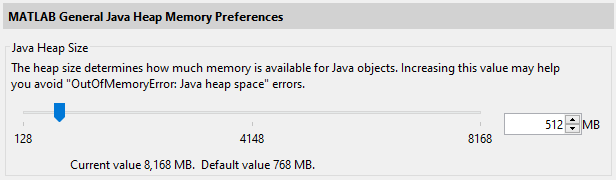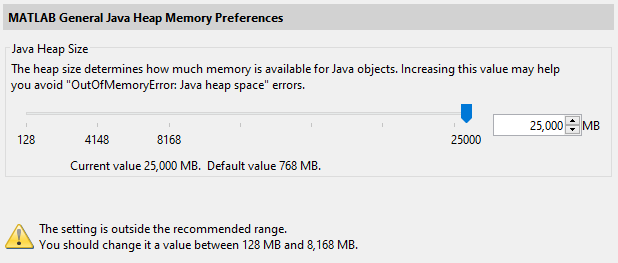# Using Bio-Formats in MATLAB¶

This section assumes that you have installed the MATLAB toolbox as instructed in the MATLAB user information page. Note the minimum recommended MATLAB version is R2017b.

As described in Using Java Libraries, every installation of MATLAB includes a JVM allowing use of the Java API and third-party Java libraries. All the helper functions included in the MATLAB toolbox make use of the Bio-Formats Java API. Please refer to the Javadocs for more information.

## Increasing JVM memory settings¶

The default JVM settings in MATLAB can result in `java.lang.OutOfMemoryError: Java heap space` exceptions when opening large image files using Bio-Formats. Information about the Java heap space usage in MATLAB can be retrieved using:

```java.lang.Runtime.getRuntime.maxMemory
```

### MATLAB Preferences (R2010a+)¶

Default JVM settings can be increased by Java Heap Memory Preferences of MATLAB (R2010a onwards) using Preferences ‣ General ‣ Java Heap Memory. We recommend to use 512 MB in general, but you don’t need to decrease the value if the default value is bigger than 512 MB.However, the maximum value allowed in the Preferences GUI (typically set to 25% of Physical Memory, whihc you can ask by the `memory` MATLAB command) can still be too small for your purpose. In that case, you can directly edit `matlab.prf` file in the folder specified by the `prefdir` MATLAB function (eg. `'C:\Users\xxxxxxx\AppData\Roaming\MathWorks\MATLAB\R2018b'`). Find the parameter `JavaMemHeapMax` in the file and increase the number that follows the capital `I` (in MB) to increase the maximum Java heap memory size. The change will be reflected by the Preferences as above.

```JavaMemHeapMax=I25000
```You’ll see a warning message as above.

### java.opts¶

Alternatively, this can also be done by creating a `java.opts` file in the startup directory and overriding the default memory settings (see this page for more information). We recommend using `-Xmx512m` (meaning 512 MB) in your `java.opts` file. Calling:

```bfCheckJavaMemory()
```

will also throw a warning if the runtime memory is lower than the recommended value.

If errors of type `java.lang.OutOfMemoryError: PermGen space` are thrown while using Bio-Formats with the Java bundled with MATLAB, you may try to increase the default values of `-XX:MaxPermSize` and `-XX:PermSize` via the `java.opts` file.

## Opening an image file¶

The first thing to do is initialize a file with the bfopen function:

```data = bfopen('/path/to/data/file');
```

This function returns an `n`-by-4 cell array, where `n` is the number of series in the dataset. If `s` is the series index between 1 and `n`:

• The `data{s, 1}` element is an `m`-by-2 cell array, where `m` is the number of planes in the `s`-th series. If `t` is the plane index between 1 and `m`:
• The `data{s, 1}{t, 1}` element contains the pixel data for the `t`-th plane in the `s`-th series.
• The `data{s, 1}{t, 2}` element contains the label for the `t`-th plane in the `s`-th series.
• The `data{s, 2}` element contains original metadata key/value pairs that apply to the `s`-th series.
• The `data{s, 3}` element contains color lookup tables for each plane in the `s`-th series.
• The `data{s, 4}` element contains a standardized OME metadata structure, which is the same regardless of the input file format, and contains common metadata values such as physical pixel sizes - see OME metadata below for examples.

### Accessing planes¶

Here is an example of how to unwrap specific image planes for easy access:

```seriesCount = size(data, 1);
series1 = data{1, 1};
series2 = data{2, 1};
series3 = data{3, 1};
% etc
series1_planeCount = size(series1, 1);
series1_plane1 = series1{1, 1};
series1_label1 = series1{1, 2};
series1_plane2 = series1{2, 1};
series1_label2 = series1{2, 2};
series1_plane3 = series1{3, 1};
series1_label3 = series1{3, 2};
```

### Displaying images¶

If you want to display one of the images, you can do so as follows:

```series1_colorMap1 = data{1, 3}{1, 1};
figure('Name', series1_label1);
if isempty(series1_colorMap1)
colormap(gray);
else
colormap(series1_colorMap1);
end
imagesc(series1_plane1);
```

This will display the first image of the first series with its associated color map (if present). If you would prefer not to apply the color maps associated with each image, simply comment out the calls to `colormap`.

If you have the image processing toolbox, you could instead use:

```imshow(series1_plane1, []);
```

You can also create an animated movie (assumes 8-bit unsigned data):

```cmap = gray(256);
for p = 1 : size(series1, 1)
M(p) = im2frame(uint8(series1{p, 1}), cmap);
end
if feature('ShowFigureWindows')
movie(M);
end
```

There are two kinds of metadata:

• Original metadata is a set of key/value pairs specific to the input format of the data. It is stored in the `data{s, 2}` element of the data structure returned by `bfopen`.
• OME metadata is a standardized metadata structure, which is the same regardless of input file format. It is stored in the `data{s, 4}` element of the data structure returned by `bfopen`, and contains common metadata values such as physical pixel sizes, instrument settings, and much more. See the OME Model and Formats documentation for full details.

To retrieve the metadata value for specific keys:

```% Query some metadata fields (keys are format-dependent)
```

To print out all of the metadata key/value pairs for the first series:

```metadataKeys = metadata.keySet().iterator();
fprintf('%s = %s\n', key, value)
end
```

Conversion of metadata to the OME standard is one of Bio-Formats’ primary features. The OME metadata is always stored the same way, regardless of input file format.

To access physical voxel and stack sizes of the data:

```omeMeta = data{1, 4};
stackSizeX = omeMeta.getPixelsSizeX(0).getValue(); % image width, pixels
stackSizeY = omeMeta.getPixelsSizeY(0).getValue(); % image height, pixels
stackSizeZ = omeMeta.getPixelsSizeZ(0).getValue(); % number of Z slices

voxelSizeXdefaultValue = omeMeta.getPixelsPhysicalSizeX(0).value();           % returns value in default unit
voxelSizeXdefaultUnit = omeMeta.getPixelsPhysicalSizeX(0).unit().getSymbol(); % returns the default unit type
voxelSizeX = omeMeta.getPixelsPhysicalSizeX(0).value(ome.units.UNITS.MICROMETER); % in µm
voxelSizeXdouble = voxelSizeX.doubleValue();                                  % The numeric value represented by this object after conversion to type double
voxelSizeY = omeMeta.getPixelsPhysicalSizeY(0).value(ome.units.UNITS.MICROMETER); % in µm
voxelSizeYdouble = voxelSizeY.doubleValue();                                  % The numeric value represented by this object after conversion to type double
voxelSizeZ = omeMeta.getPixelsPhysicalSizeZ(0).value(ome.units.UNITS.MICROMETER); % in µm
voxelSizeZdouble = voxelSizeZ.doubleValue();                                  % The numeric value represented by this object after conversion to type double
```

To convert the OME metadata into a string, use the `dumpXML()` method:

```omeXML = char(omeMeta.dumpXML());
```

## Changing the logging level¶

By default, `bfopen` uses `bfInitLogging` to initialize the logging system at the WARN level. To change the root logging level, use the DebugTools methods as described in the Logging section.

```% Set the logging level to DEBUG
loci.common.DebugTools.setRootLevel('DEBUG');
```

## Reading from an image file¶

The main inconvenience of the bfopen.m function is that it loads all the content of an image regardless of its size.

```reader = bfGetReader('path/to/data/file');
```

You can then access the OME metadata using the `getMetadataStore()` method:

```omeMeta = reader.getMetadataStore();
```

Individual planes can be queried using the bfGetPlane.m function:

```series1_plane1 = bfGetPlane(reader, 1);
```

To switch between series in a multi-image file, use the setSeries(int) method. To retrieve a plane given a set of (z, c, t) coordinates, these coordinates must be linearized first using getIndex(int, int, int)

```% Read plane from series iSeries at Z, C, T coordinates (iZ, iC, iT)
% All indices are expected to be 1-based
iPlane = reader.getIndex(iZ - 1, iC -1, iT - 1) + 1;
```

## Saving files¶

The basic code for saving a 5D array into an OME-TIFF file is located in the bfsave.m function.

For instance, the following code will save a single image of 64 pixels by 64 pixels with 8 unsigned bits per pixels:

```plane = zeros(64, 64, 'uint8');
bfsave(plane, 'single-plane.ome.tiff');
```

And the following code snippet will produce an image of 64 pixels by 64 pixels with 2 channels and 2 timepoints:

```plane = zeros(64, 64, 1, 2, 2, 'uint8');
bfsave(plane, 'multiple-planes.ome.tiff');
```

By default, `bfsave` will create a minimal OME-XML metadata object containing basic information such as the pixel dimensions, the dimension order and the pixel type. To customize the OME metadata, it is possible to create a metadata object from the input array using createMinimalOMEXMLMetadata.m, add custom metadata and pass this object directly to `bfsave`:

```plane = zeros(64, 64, 1, 2, 2, 'uint8');
pixelSize = ome.units.quantity.Length(java.lang.Double(.05), ome.units.UNITS.MICROMETER);
pixelSizeZ = ome.units.quantity.Length(java.lang.Double(.2), ome.units.UNITS.MICROMETER);
```

The dimension order of the file on disk can be specified in two ways:

• either by passing an OME-XML metadata option as a key/value pair as shown above
• or as an optional positional argument of `bfsave`

If a metadata object is passed to `bfsave`, its dimension order stored internally will take precedence.

Initializing a Bio-Formats reader can consume substantial time and memory. Most of the initialization time is spend in the setId(java.lang.String) call. Various factors can impact the performance of this step including the file size, the amount of metadata in the image and also the file format itself.

One solution to improve reading performance is to use Bio-Formats memoization functionalities with the loci.formats.Memoizer reader wrapper. By essence, the speedup gained from memoization will only happen after the first initialization of the reader for a particular file.

The simplest way to make use the `Memoizer` functionalities in MATLAB is illustrated by the following example:

```% Construct an empty Bio-Formats reader
% Decorate the reader with the Memoizer wrapper
r = loci.formats.Memoizer(r);
% Initialize the reader with an input file
% If the call is longer than a minimal time, the initialized reader will
% be cached in a file under the same directory as the initial file
% name .large_file.bfmemo
r.setId(pathToFile);

% Perform work using the reader

r.close()

% If the reader has been cached in the call above, re-initializing the
% reader will use the memo file and complete much faster especially for
% large data
r.setId(pathToFile);

r.close()
```

If the time required to call setId(java.lang.String) method is larger than DEFAULT_MINIMUM_ELAPSED or the minimum value passed in the constructor, the initialized reader will be cached in a memo file under the same folder as the input file. Any subsequent call to `setId()` with a reader decorated by the `Memoizer` on the same input file will load the reader from the memo file instead of performing a full reader initialization.

More constructors are described in the Memoizer javadocs allowing to control the minimal initialization time required before caching the reader and/or to define a root directory under which the reader should be cached.

As Bio-Formats is not thread-safe, reader memoization offers a new solution to increase reading performance when doing parallel work. For instance, the following example shows how to combine memoization and MATLAB parfor to do work on a single file in a parallel loop:

```% Construct a Bio-Formats reader decorated with the Memoizer wrapper
% Initialize the reader with an input file to cache the reader
r.setId(pathToFile);
r.close()

nWorkers = 4;

% Enter parallel loop
parfor i = 1 : nWorkers
% Initialize logging at INFO level
bfInitLogging('INFO');
% Initialize a new reader per worker as Bio-Formats is not thread safe